## Excel TIMEVALUE Function

Supposing there is a text formatting time list in your worksheet, how can you convert the time list to proper time? Actually the TIMEVALUE function can help you solve the problem. This article provides syntax and detailed sample of TIMEVALUE function to help you easily use it in Excel.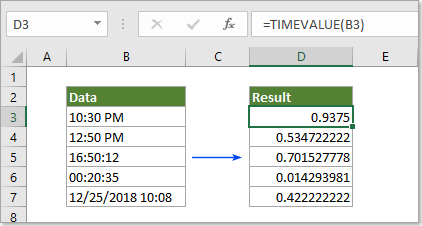Description of TIMEVALUE function

Syntax of TIMEVALUE function

Arguments of syntax

Usage of TIMEVALUE function

#### Description of TIMEVALUE function

The Microsoft Excel TIMEVALUE function can help to return decimal number of a time which was formatted as text string.

#### Syntax of TIMEVALUE function

=TIMEVALUE(time_text)

#### Arguments of syntax

• Time-text: The text formatting of a time or datetime which can be recognized by Excel.

1) Directly reference to a time or date and time cell which formatted as text: =TIMEVALUE(B3).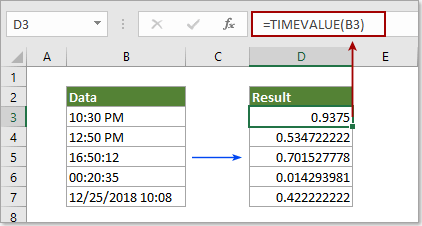2) Directly enter time as text string and embraced with quotation marks: =TIMEVALUE("10:30 PM").

Or =TIMEVALUE("25-Dec-2018 10:30 PM") for date and time text format.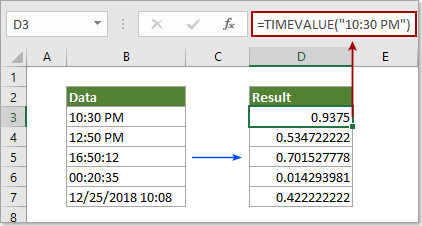#### Usage of TIMEVALUE function

This section will show you detailed steps to use the TIMEVALUE function in Excel.

1. Select a cell you want to output the time result, enter formula =TIMEVALUE(B3) into the Formula Bar and press the Enter key. See screenshot: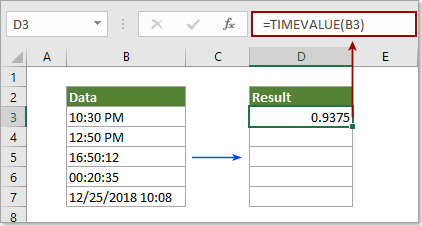Then drag the Fill Handle down to apply the formula to other cells as below screenshot shown.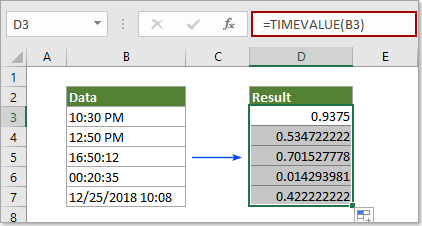Remark: #VALUE! will occur if the referenced time cannot be recognized as a valid time by Excel.